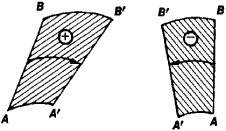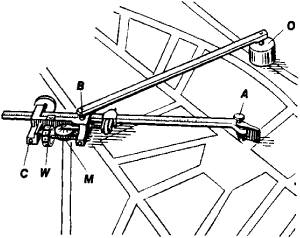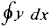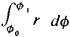# Planimeter

Also found in: Dictionary, Thesaurus, Medical, Wikipedia.

## planimeter

[plə′nim·əd·ər]
(engineering)
A device used for measuring the area of any plane surface by tracing the boundary of the area.
McGraw-Hill Dictionary of Scientific & Technical Terms, 6E, Copyright © 2003 by The McGraw-Hill Companies, Inc.
The following article is from The Great Soviet Encyclopedia (1979). It might be outdated or ideologically biased.

## Planimeter

a mathematical instrument for measuring the areas of plane figures of arbitrary shape. The most widespread type is the polar planimeter, which was invented by the Swiss mathematician J. Amsler in 1854.Figure 1

The principle of operation of such a planimeter is shown in Figure 1. When the oriented line segment AB moves in the plane, the area of the figure swept out by it is equal to the product of the length of AB and the length of the arc described by the segment’s midpoint. The quantity σ is equal to the length of the arc of rotation of the integrating wheel if the wheel is placed at the midpoint of AB and its axis coincides with the segment. The polar planimeter (Figure 2) has a polar arm and a tracing arm, which are pivoted at the point B. The polar arm can be rotated about the pole pivot O. The integrating wheel and the counting mechanism are located on a special carriage that can slide along the tracing arm, thereby changing the length of the arm.Figure 2. Polar planimeter: (O) pole, (OB) polar arm, (A) tracing pointer, (AB) tracing arm, (C) carriage, (W) integrating wheel, (M) counting mechanism

In every planimeter the function of the sweeping line segment is performed by the tracing arm, at one end of which is fastened a pointer for tracing out the figure’s outline and the other end of which moves along a “directrix.” The directrix is a straight line in a linear planimeter, a circle in a polar planimeter, and a point in a radial planimeter. Linear and polar planimeters are used to compute areas bounded by curves given graphically and to calculate integrals of the formRadial planimeters permit integrals of the formto be found if the curve r = f (ϕ) is drawn in polar coordinates.

## planimeter

A mechanical integrator for measuring the area of a plane surface usually within a given perimeter on a map.
McGraw-Hill Dictionary of Architecture and Construction. Copyright © 2003 by McGraw-Hill Companies, Inc.
References in periodicals archive ?
A planimeter method for the determination of the percentage composition of rocks.
Areas have been calculated by means of planimeters. Following planimeters were used: of Fallon type, Posemer type, and later the thread planimeter of Alder type.
The heat of fusion was determined by measuring the area of the fusion endotherm with a planimeter and by using indium (specific heat of fusion = 6.8 J/g) as a calibration sample.
We calculated the mean area of the trichome wing in each species considering 40 samples with a Digital Planimeter KP-80 Placam Koizumi.
Estimators traditionally calculate distance and area measurements on a landscape plan by using a scale (architect or engineer), measuring wheel, and planimeter. Scales are used to measure distances and lengths on drawings.
The areas [A.sub.1], [A.sub.2], ..., [A.sub.n] can be determined by using a digitizer or a planimeter.
Analysis was limited to measuring areas with a planimeter and adding them up.
As the sample froze, the signal from the calorimeter was recorded on a strip chart recorder and areas under curves were measured using a hand-held planimeter. The average of three measurements (less than 3% variation was observed between measurements) was used in all calculations.
The area of each trace was then measured with a planimeter. The remaining bark was then calculated as a percentage of total log area without bark.

Site: Follow: Share:
Open / Close